# Topological Quantum Computing

What is topological quantum computing, where is the field heading into, what is the current state of the art research…In this blog, which was part of UC Berkeley’s Quantum Computing Course with Umesh Vazirani with my classmates Andre He, Dennis Feng, Jeffrey Zhang, we attempted to answer all these questions. To us, quantum computing was the reason why we took the course, but topological quantum computing had such elegant mathematics in the backdrop that was super intellectually stimulating.

This review project was done in Spring 2017. I do not know of any major paradigm shift from 2017 until now, May 2019. But I would love to hear more about what has been advancing in the field! If you like this blog and there is something new you would like to share, please feel free to reach out!

# Introduction

Quantum computing exploits quantum-mechanical phenomena such as superposition and entanglement to perform operations on data, which in many cases, are infeasible to do efficiently on classical computers. The basis of this data is the qubit, which is the quantum analog of the classical bit. Many of the current implementations of qubits, such as trapped ions and superconductors, are highly susceptible to noise and decoherence because they encode information in the particles themselves. Topological quantum computing seeks to implement a more resilient qubit by utilizing non-Abelian forms of matter to store quantum information. In such a scheme, information is encoded not in the quasiparticles themselves, but in the manner in which they interact and are braided. Thus, this scheme is topological, meaning that only the macroscopic topological properties of the particles’ worldlines matter. This makes topological quantum computing more resistant to decoherence and noise issues which plague other qubit implementations.

In topological quantum computing, qubits are initialized as non-abelian anyons, which exist as their own antiparticles. Then, operations (what we may think of as quantum gates) are performed upon these qubits through “braiding” the worldlines of the anyons. Because of the non-Abelian nature of these particles, the manner in which they are exchanged matters (similar to noncommutativity). Another important property of these braids to note is that local perturbations and noise will not impact the state of the system unless these perturbations are large enough to create new braids. Finally, a measurement is taken by fusing the particles. Because anyons are their own antiparticles, the fusion will result in the annihilation of some of the particles, which can be used as a measurement.

Currently, topological quantum computing is inching towards becoming a reality, with one of the biggest milestones — observing the quasiparticles which form the basis of topological quantum computing — winning the Nobel Prize in 2016. With much of the theoretical framework having been developed in the past 20 years, the experimental progress recently has been accelerating to implement the theories.

# Theory

First, we need to briefly define a few key concepts. Quantum Computation is any computational model based upon the theoretical ability to manufacture, manipulate and measure quantum states. A topological quantum computer is a device that relies upon topologically ordered states of matter to carry out the fault-tolerant quantum computation. A system is in a topological phase if, at low temperatures and energies and long wavelengths, all observable properties such as correlation functions are invariant under smooth deformation of the space-time manifold in which the system lives.

1. Anyons

In topological quantum computing, a qubit is composed of a group of anyons, which do not appear in 3D systems. In 3D, every particle is either a fermion or a boson, and exchanging two particles bring up an overall sign, either + or -:

But in 2D, particles obey exchange statistics

for any complex phase θ. These particles are defined as anyons. Mathematically, this is related to the fact that the group of motions of two points in 3-dimensional space is S2, a group of order 2, whereas in 2-dimensional space the group of motions of n points in a disk is an infinite group. This suggests that exchange statistics of point-like particles in 2 spatial dimensions are richer than in 3 dimensions.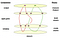Figure 1. A topological quantum computation scheme, showing the braided worldlines of several anyons.

Once we assume that we can manufacture, manipulate and measure 2-dimensional topological phases of matter per the requirement of quantum computing, we have a theoretical scheme for topological quantum computation. As Figure 1 shows , particle-antiparticle pairs are drawn from the vacuum to initiate the states. The vertical direction is the time axis and each cross-section surface with labeled points are associated with a Hilbert space at a certain time. Particle exchange, called braiding, corresponds to a unitary operation on the associated Hilbert space. In other words, braiding is equivalent to applying a quantum gate to the qubit states.

Suppose our system supports exactly r distinguishable nondecomposable anyon types, which we label by a set L = {0, . . . , r — 1} of “colors” or “particle types”. We include the vacuum as a sort of invisible anyon type which we label with a 0. Anyons on a surface are point-like so we can see them as small boundary circles on the surface. As we interpret the anyons as (quasi)-particles, each anyon a must have an antiparticle. A convenient topological interpretation of antiparticles is that a positively oriented circle labeled by a is the same as a negatively oriented circle labeled by a+. Topological invariance means that any topologically allowed operations such as anyon exchange, or twisting part of a surface, must correspond to a unitary operator on the corresponding Hilbert space.

To measure the qubit states after we perform a computation, we fuse, or bring together, the anyons and we measure the fusion outcomes. The fusion outcomes (for example, whether or not the particles annihilate each other) depends on the quantum states of the qubits.

So the process of computation goes as follows: 1) create particle-antiparticle pairs to initialize our qubits, 2) braid them together to perform gate operations, and 3) measure the fusion outcomes. One key point is that after braiding the particles’ world lines, a neighboring particle-antiparticle pair may have obtained a different total charge (i.e. the label). Slight variations in the process such as small deviations in the particle’s worldline, perhaps due to noise, do not influence the output. To get meaningful information we must repeat the same process several times, taking a tally of the outputs.

It has been proven that there are finitely many possible models of anyonic systems with exactly n distinct particle types. One possible theory when n=3 is the Ising theory in which the famous and simplest non-abelian anyons are predicted. Ettore Majorana showed in 1937 that the complex Dirac equation can be separated into a pair of real wave equations, each of which describes a real fermionic field without the distinction of particle and antiparticle. The decomposition of a complex Dirac fermion into a superposition of two real Majorana fermions is a formal step without physical consequences. But Majorana suggested that neutral particles might be described by a single real field and concluded that “there is now no need to assume the existence of antineutrons or antineutrinos”. We now know that the neutron and antineutron are distinct particles, but the neutrino and antineutrino could well be the same particle observed in different states of motion.

The electron and hole excitations of the superconductor play the role of particle and antiparticle. Electrons (filled states at energy E above the Fermi level) and holes (empty states at E below the Fermi level) have opposite charge, but the charge difference of 2e can be absorbed as a Cooper pair in the superconducting condensate. At the Fermi level (E=0, in the middle of the superconducting gap), the eigenstates are charge-neutral superpositions of electrons and holes. Those midgap excitations of a superconductor are Majorana fermions.

Much of the recent excitement followed after Liang Fu and Charles Kane showed that conventional spin-singlet, s-wave superconductivity could be used, in combination with the strong spin-orbit coupling of a topological insulator.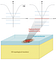Figure 2: A scheme of realizing Majorana fermions in a 2D system. The Majorana fermions form in vortices created on the superconductor surface in the diagram.

The basic mechanism is illustrated in Figure 2 . The three-dimensional (3D) topological insulator has an insulating bulk and a metallic surface. The 2D surface electrons are massless Dirac fermions, very much like in graphene, but without the spin and valley degeneracies of graphene. A superconductor deposited on the surface opens an excitation gap, which can be closed locally by a magnetic field. The magnetic field penetrates as an Abrikosov vertex, with subgap states En = (n+a)δ, where n are integers, are level spacings determined by the superconducting gap and Fermi energy, and a are either 0 or ½ required by the electron-hole symmetry. For a=0 and n=0, the E0=0 zero mode would be a Majorana fermion.

Majorana fermions (MFs) can be created in a variety of ways. The requirement is to take a superconductor, split the electron spin degeneracies (for example through spin-orbit or Zeeman coupling), and close and reopen the superconducting gap . There are several candidate 2D systems that can physically realize these MFs, including the ν= 5/2 fractional quantum Hall state, chiral p-wave superconductors, topological insulators, and semiconductor heterostructures. However, it turned out that MFs were perhaps more easily created in a 1D system , as described below.

# Experiment

The first widely regarded experimental observation of MFs occurred in the interface between a superconductor and a semiconductor 1D nanowire . The experimenters placed a superconducting electrode and a normal metal electrode at either end of an InSb nanowire, and subjected the nanowire to a magnetic field pointing parallel to its axis, as shown in Figure 3. The InSb nanowire was chosen mainly because of its high spin-orbit interaction. Additionally, the Zeeman effect (from the magnetic field) and the spin-orbit coupling were important to break the electron spin degeneracies in the nanowire. As the experimenters increased the magnetic field, the excitation gap in the superconducting nanowire closed and reopened, which should have created so-called “Majorana zero modes” at the ends of the wire. By measuring the differential conductance of the nanowire through this magnetic field sweep, they observed a peak at zero voltage, as shown in Figure 4. Under the conditions of the experiment, this peak could only have been explained by the Majorana zero modes causing current to flow through the wire. Thus, they concluded that they had detected Majorana fermions.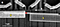Figure 3: SEM image of the superconducting (S) and normal (N) electrodes in contact with the InSb nanowire. There is a magnetic field (B) that points parallel to the nanowire.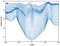Figure 4: Plots of differential conductance (dI/dV) vs. voltage, at a temperature of 70 mK. The curves are the plots at increasing B fields (from 0 to 490 mT, in 10 mT intervals), and all except the B = 0 curve are offset for clarity.

The next experimental question to ask is: how do we braid these anyons around each other? (Recall that braiding corresponds to applying a unitary gate on the qubits.) In the setup described earlier with two MFs at either end of a nanowire, how can we swap their positions without having them fuse? Alicea et al.  described a method to accomplish this, as shown in Figure 5. First, one attaches a perpendicular nanowire to form a T-junction. Then, using precisely controlled electric fields, one can transport one of the MFs onto the bottom of the T, slide the second MF across the T, and transport the first MF to the opposite side. By having a network of these T-junctions, one can perform arbitrary braid operations on the MFs.

# Error Correction with Topological Quantum Computing

As mentioned earlier in this study, we noted that the reason why topological quantum computing is so appealing is that the local perturbations and noise are less likely to affect the state of the system, meaning it is less likely for decoherence to occur. This is mathematically stated by the threshold theorem. The threshold theorem states that given that the probability of a quantum error per gate is low enough (lower than a threshold value pc), we are able to use the system for quantum computation for an indefinite amount of time with accuracy. Traditionally, the values of pc needed are quite low, on the order of 10–5. However, with topological quantum computing using error correction, the values of pc are on the order of 10–2 . In 2012, Yao et al have been able to experimentally show topological error corrections to work in cluster state computing.

The group used six photons structured in a 3D cell structure known as a “cell complex” that has redundant topological correlations. An illustration of the cell complex is shown in Figure 6. These correlation values are always one in an undisturbed system and under specific errors, follow the scheme described by the table in Figure 7. Through this correlation scheme, we can determine if there is an error and on what qubit the error occurs on.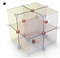Figure 6: Photons in this cluster state have topological properties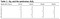Figure 7: Redundancy of information coming from the cluster state allows us to observe errors when the correlations are off

Running the experiments on an intricate system of lasers gives us the resulting expectation values that confirm the theoretical understanding of the topological structure. The graph in Figure 8 shows the experimental results.

# Current State of the Art Research

Currently, there are many institutions that are betting on topological quantum computing on becoming realized and they are putting a lot of resources in doing so. One group, in particular, is Microsoft’s Station Q, a group dedicated to research in this field. They have many sites that are working on separate yet interconnected pieces of the topological quantum computing puzzle. The teams are listed below.

● Santa Barbara: Founded by Dr. Michael Freedman, a Field Medal winner. Researching the topological phases of matter, specifically Majorana zero modes. Practical implementation of topological qubits.

● Delft: Testing the stability of topological quantum gates and working on the demonstration of the failure of decoherence in topologically protected states.

● Redmond: Developing real-world quantum algorithms and understanding their implications, as well as designing software architecture.

● Sydney: Addressing the challenges of scaling up quantum computing. Interfacing between quantum systems and working on classical control and readout.

● Copenhagen: Studying the use of and properties of the Majorana fermions and building systems that can support such a particle.

● Purdue: Studying quantum mechanical properties of electrons in ultra-high purity III-V semiconductor devices. Heavily focused on molecular beam epitaxy (MBE), which allows them to build structures one atomic layer at a time.

● ETH Zurich: Looking for the right materials that permit quantum computers to exist.

Recently, researchers from Zurich, Intel, and Redmond published a paper that described emulation of quantum circuits on classical machines to test theoretical quantum algorithms. The emulations are much faster in performance than simulations of quantum circuits. Current simulations compute slow, large matrix-vector products in computing each quantum gate in a classical computer. Instead, the emulations reduce the amount of complicated linear algebra and rely on using classical algorithms, such as the fast Fourier transform, to run the quantum programs .

Currently, the field of topological quantum computation is still in its infancy, compared to more conventional quantum computation, such as with trapped ions or with superconducting circuits. However, the recent incremental progress, both in the theoretical and experimental aspects, indicates that the future of the field is promising.

Acknowledgment

Alexander Shengzhi Li, Andre He, Dennis Feng, Jeffrey Zhang worked on this study together as part of a quantum computing course at the University of California, Berkeley Computer Science Department with Professor Umesh Vazirani. The study would not be completed without the feedback of the professor or the class TAs.

References

1. Collins, G. P. (2006). Computing with quantum knots. Scientific American, 294(4), 56–63.
2. Beenakker, C. W. J. (2013). Search for Majorana fermions in superconductors. Annu. Rev. Condens. Matter Phys., 4(1), 113–136.
3. Alicea, J., Oreg, Y., Refael, G., von Oppen, F., Fisher, M. P. A. (2010). Non-Abelian statistics and topological quantum information processing in 1D wire networks. arXiv preprint arXiv:1006.4395v2.
4. Mourik, V., Zuo, K., Frolov, S. M., Plissard, S. R., Bakkers, E. P. A. M., & Kouwenhoven, L. P. (2012). Signatures of Majorana fermions in hybrid superconductor-semiconductor nanowire devices. Science, 336(6084), 1003–1007.
5. Yao, X. C., Wang, T. X., Chen, H. Z., Gao, W. B., Fowler, A. G., Raussendorf, R., et al. (2012). Experimental demonstration of topological error correction. Nature, 482(7386), 489–494.
6. Haner, T., Steiger, D.S., Smelyanskiy, M., Troyer, M. (2016). High Performance Emulation of Quantum Circuits. arXiv preprint arXiv:1604.06460v1.

Written by